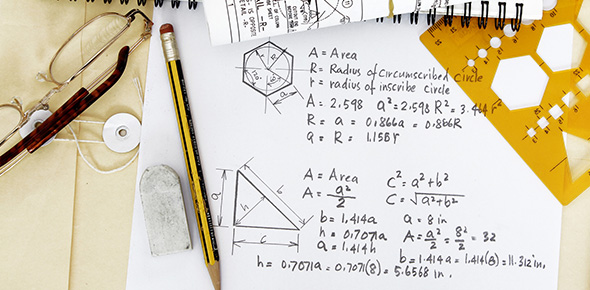# Area And Circumference Of Circles Quiz

5 QuestionsSettingsRelated Topics
• 1.
The area is the space  inside of the circle.
• A.

True

• B.

False

• 2.
The parts of the circle are:
• A.

• B.

Diameter

• C.

Circle

• 3.
What is the formula for finding the diameter?
• A.

D=1/2d

• B.

D=2r

• C.

D=2piR

• 4.
What is the formula for finding the radius?
• A.

R=2D

• B.

R=half of D

• C.

D=axbxc

• 5.
What is the diameter of circle?